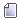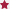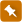###Author Topic: Each Surface-Layout, Each Graph of a Mathematical Equation that Elucidates  (Read 9365 times)

#### Parker Emmerson

• We are only beginning to understand what the meaning of the, phenomenological velocity, solution truly is and how the curvatures that result from the solutions to the v-variable are effecting the perceived phenomena in our reality
• Newbie
••• Posts: 8
• Karma: +0/-0
• Phenomenological Cone Structures and Mathematics##### Each Surface-Layout, Each Graph of a Mathematical Equation that Elucidates
« on: September 18, 2016, 07:44:27 PM »
Each Surface-Layout, Each Graph of a Mathematical Equation that Elucidates the Nature of Reality Can Be Thought of as A Vajra - An Object of Meditation.

https://en.wikipedia.org/wiki/Vajra

People speak of Energy to describe the phenomenon of that which is neither created nor destroyed, but really, all that is needed to describe that phenomenon is contained within the, phenomenological velocity, equation, also known as V-Curvature.